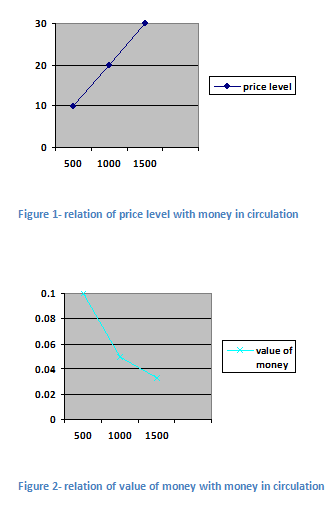# Quantity theory of money

theory by Irving Fisher

According to quantity theory of money if the money in circulation is increased, the price level also rises. The percentage or proportion of rise in price level is just equal to percentage or proportion of increase in money in circulation. The price level has direct proportional relation with money in circulation. Here money in circulation means amount of currency issued by monetary authority. Since, the value of money is inversely related to price level, it decreases with increase in money in circulation and vice versa.

Symbolically,

If M ↑ P ↑ but value of money ↓
If M ↓ P but ↓ value of money ↑

To explain this theory, Irving Fisher has used an equation of transaction the equation is

MV = PT (or MV=PQ)

Where,
M= money in circulation
V= velocity of money in circulation
P= price level

Velocity of money in circulation is the number of times the money in circulation is revolved in a year. The multiple of M and V gives the total supply of money and the multiple of P and T gives total demand for money. According to fisher, if M is increased arbitrarily by monetary authority by a certain percent or proportion the P too rises by equal percent or proportion. The V and T remain constant. The change in M brings change only in P.

This theory can be explained with the help of table and figure as following

 Money in circulation (M) Price level (P) Value of re. 1 (1/P) Rs 500 billions Rs 10 0.1 Rs 1000 billions Rs 20 0.05 Rs 1500 billions Rs 30 0.033

In the above table, when money in circulation is increased from Rs 500 billions to Rs 1000 billions and Rs 1500 billions, the price level is increased from Rs 10 to Rs 20 and Rs 30 respectively. It shows the direct proportional relationship between price level and money in circulation. If we represent price level with respect to money in circulation we obtain an upwardly sloped straight line from origin. In the above table value of re 1 is decreased from 0.1 units to 0.05 and 0.033 units when the money in circulation is increased from Rs 500 billions to Rs 1000 billions and Rs 1500 billions respectively. if we represent value of re 1 with respect to M we obtain a monotonically downwardly sloped curve as shown below:In the above first figure the curve liner in shape and upwardly sloped from origin represents direct proportional relationship between price level and money in circulation. In the second figure relationship between value of money and money in circulation is shown by convex curve.

In the above Fisher’s equation bank money is not included therefore the equation is reformed into
MV +M’V’ = PT
Where
M’= bank money
V’ = velocity of bank money’

Assumptions

1. Money is a neutral factor. Change in money in circulation doesn’t bring change in real factors like investment, production, volume of trade and employment etc.
2. Money is demanded only to buy and sells the goods and services.
3. M is arbitrarily determined by monetary authority regardless the economic situation.
4. P is passive factor because it doesn’t affect the volume of money in circulation, volume of trade, employment etc.
5. V and T are independent of M and P and are constant.
6. P is directly proportional to M.

Criticisms

1. Money is not neutral factor. Change in money in circulation brings change in real factors like investment, production, volume of trade and employment etc.
2. Money is not demanded only to buy and sells the goods and services.
3. M is not arbitrarily determined by monetary authority.
4. P is not passive factor. It is active because it affects the volume of money in circulation, volume of trade, employment etc.
5. V and T are not independent of M and P and are not constant.
6. P is not directly proportional to M.